Course Content

Classification with Python

##Splitting the Nodes

During the training, we need to find the best split at each Decision Node. When we split the data into two nodes, we want different classes to be in other nodes.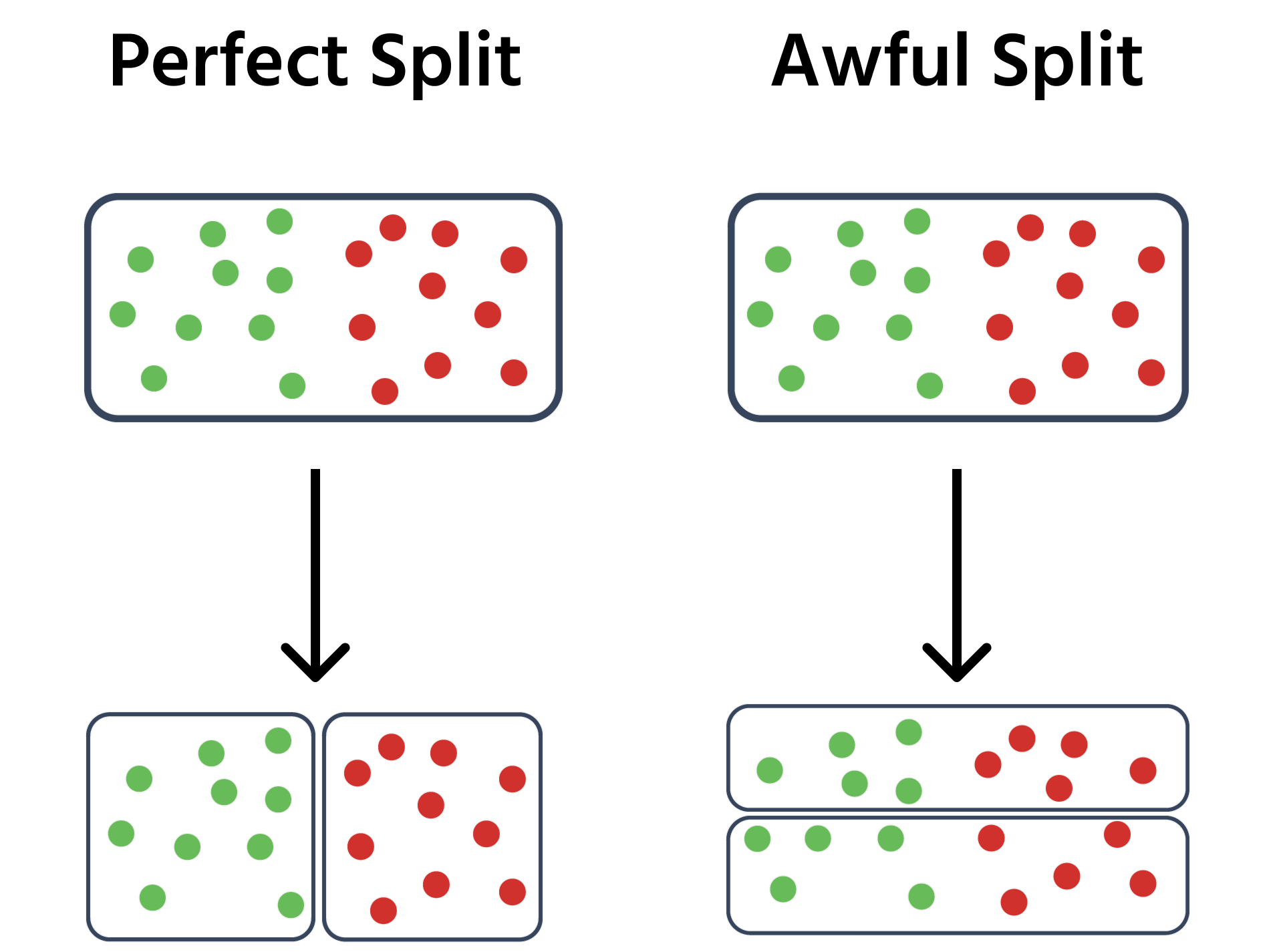• Best case scenario: All data points in a node are of the same class.
• Worst case scenario: Equal number of data points for each class.

To measure how good a split is, we can calculate the Gini Impurity.
It is a probability that if we randomly take two points from a node(with replacement), they will be different classes. The less this probability(impurity), the better split.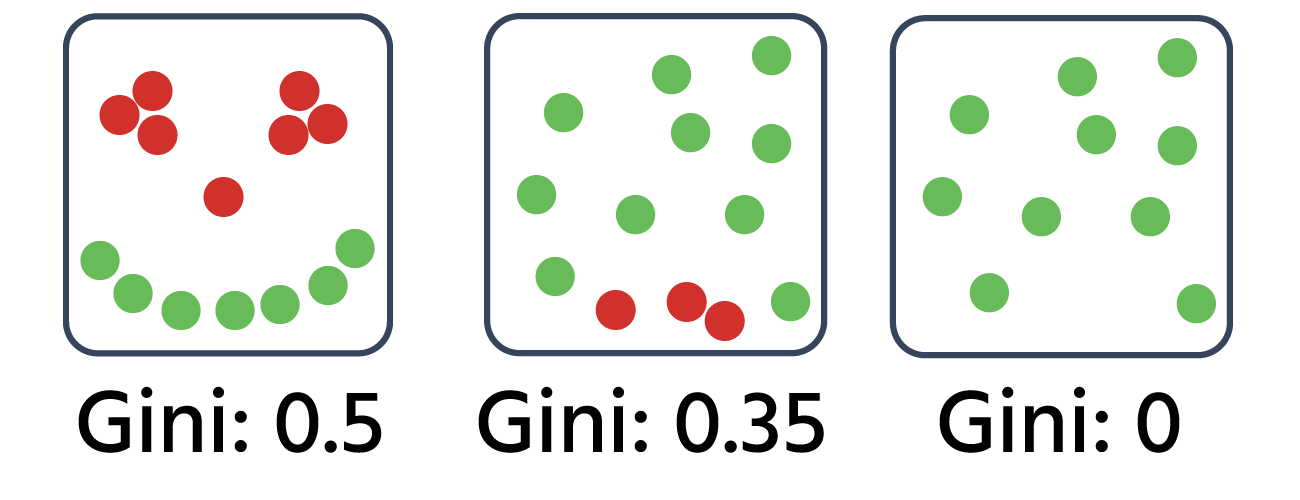You can calculate the Gini impurity for binary classification using following formula: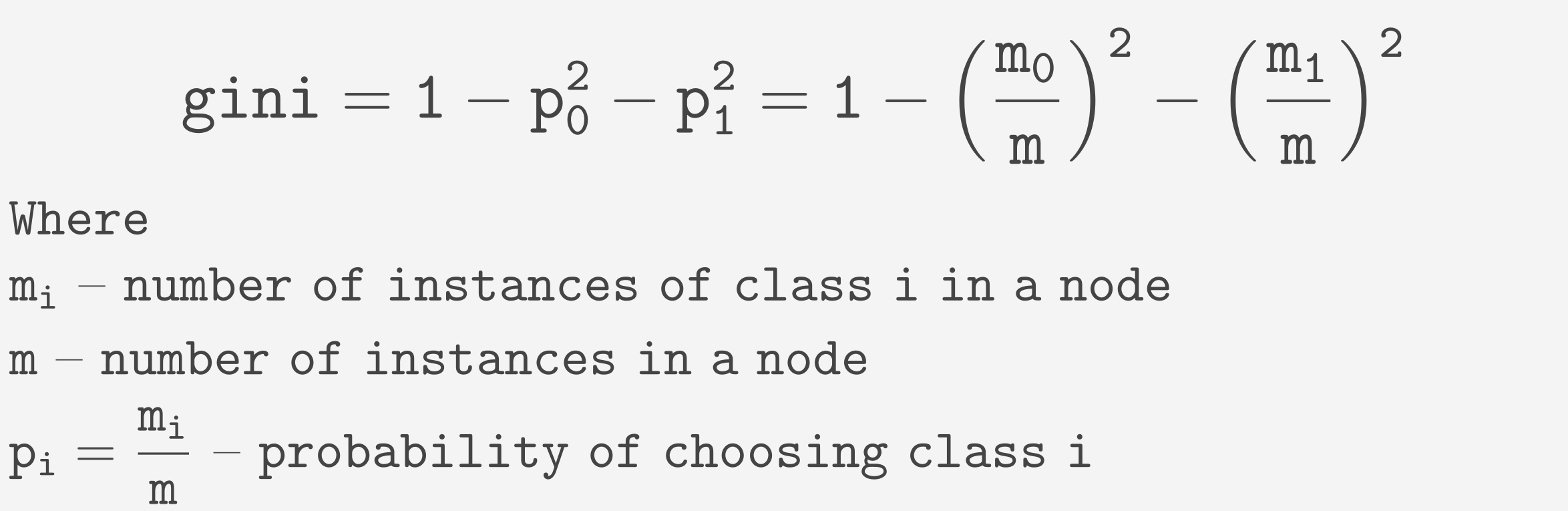And for multiclass classification the formula is: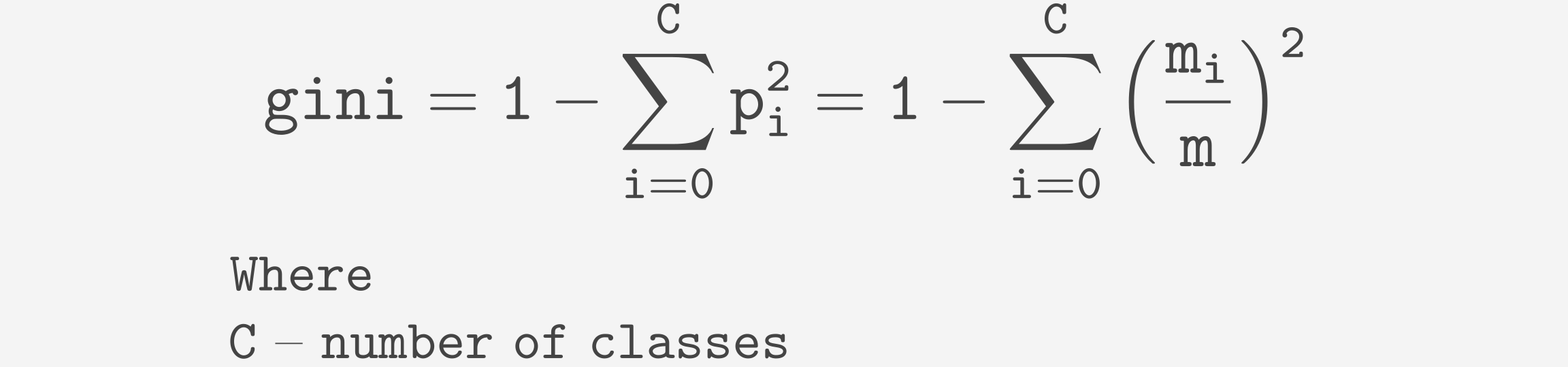We can measure how good the split is by taking the weighted sum of gini scores for both nodes obtained from a split. That's the value we want to minimize.
To split a Decision Node, we need to find a feature to split on and the threshold.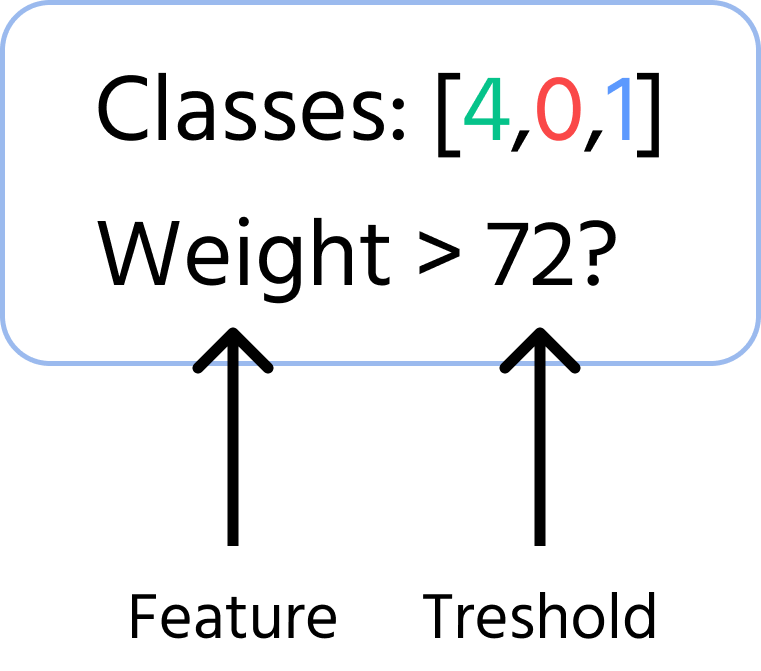At a Decision Node, the algorithm greedily finds the best threshold for each feature. Then it chooses the split with the lowest gini impurity out of all features(if there is a tie, it chooses randomly).